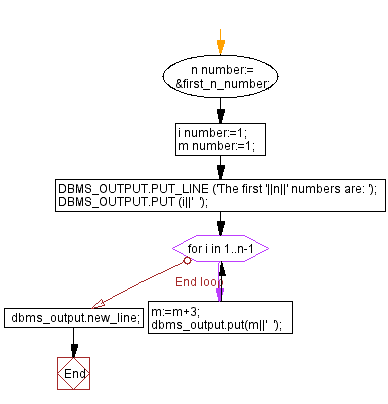﻿ PL/SQL Control Statement: Print 1st n numbers with a difference of 3 and starting from 1 - w3resource# PL/SQL Control Statement Exercises: Print 1st n numbers with a difference of 3 and starting from 1

## PL/SQL Control Statement: Exercise-24 with Solution

Write a program in PL/SQL to print 1st n numbers with a difference of 3 and starting from 1.

Sample Solution:

PL/SQL Code:

``````DECLARE
n number:= &first_n_number;
i number:=1;
m number:=1;
BEGIN
DBMS_OUTPUT.PUT_LINE ('The first '||n||' numbers are: ');
DBMS_OUTPUT.PUT (i||'  ');
for i in 1..n-1 loop
m:=m+3;
dbms_output.put(m||'  ');
END LOOP;
dbms_output.new_line;
END;
/
```
```

Flowchart:Sample Output:

```Enter value for first_n_number: 10
old   2:   n number:= &first_n_number;
new   2:   n number:= 10;
The first 10 numbers are:
1  4  7  10  13  16  19  22  25  28

PL/SQL procedure successfully completed.```

Improve this sample solution and post your code through Disqus

What is the difficulty level of this exercise?

﻿